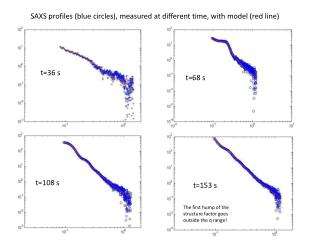DownloadDownload Presentationt=36 s

# t=36 s

Télécharger la présentation## t=36 s

- - - - - - - - - - - - - - - - - - - - - - - - - - - E N D - - - - - - - - - - - - - - - - - - - - - - - - - - -
##### Presentation Transcript

1. SAXS profiles(bluecircles), measured at differenttime, withmodel (redline) t=36 s t=68 s t=108 s t=153 s The first humpof the structurefactorgoesoutside the q range!

2. Data have been modelled by: where: S(q,a,µ) is the Percus-Yevick structure factor for given inter-particles correlation distance a on a volume fraction µ, P(R) is the log-normal size (Rm) distribution Φ(qR) is the form factor of a single spherical particle. Furthermore, C is a scale factor, N is proportional to the scattering particles and the power law term A/qβ t=270 s Structurefactorisnownegligible…humps end up outside the q range!! The fitparameters are: A, b, CN, a, m, Rm, DRm